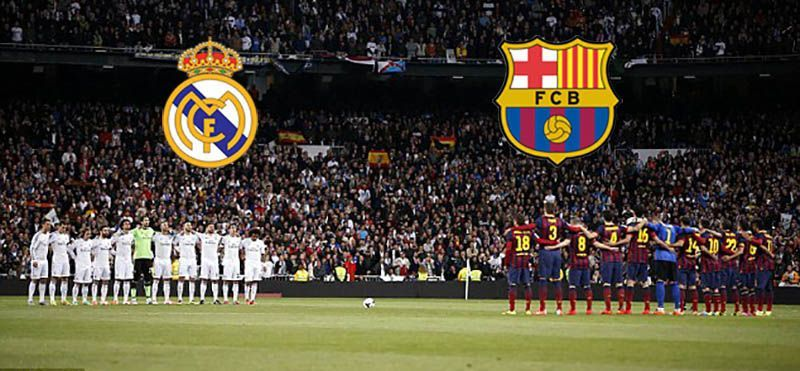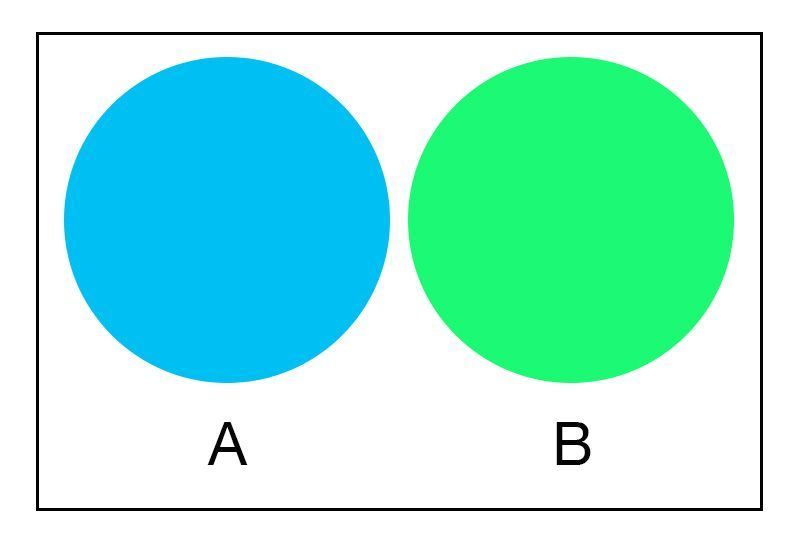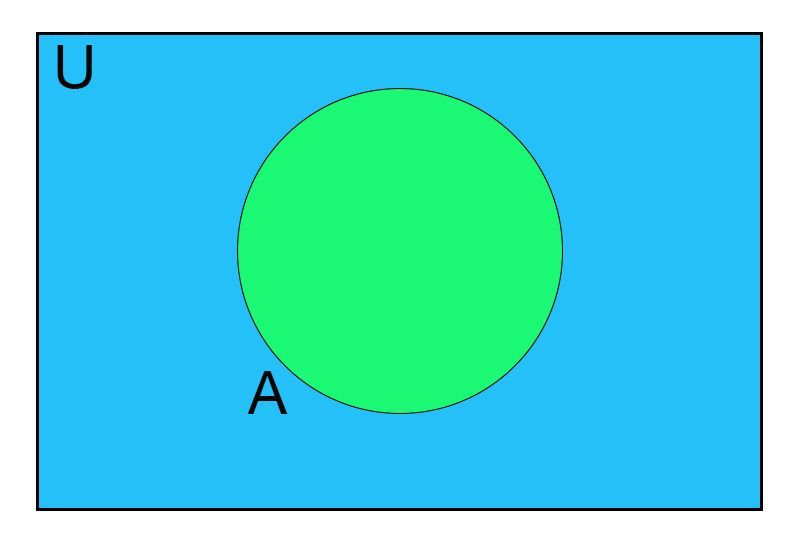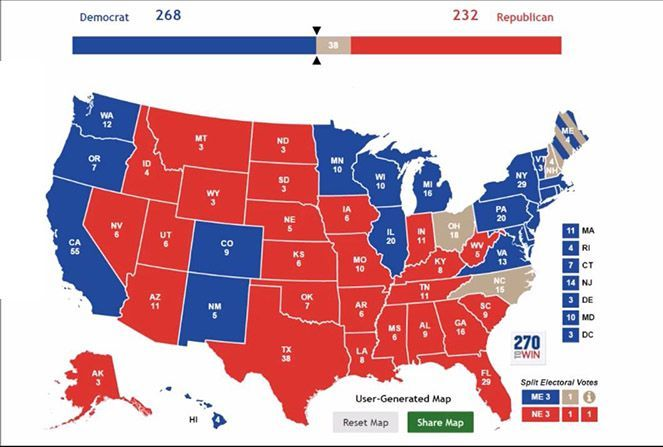# Properties of Sets# Universal Sets

A set which contains all the sets under consideration is called the Universal set.

A rectangle is used to represent the universal set.# Subset

If every element of set A is in set B, then A is said to be the subset of B.

The symbol ⊆ is used to denote subset.

# Superset

If A is a subset of B then we can say that B is the superset of A as it contains all the elements of A.

The symbol ⊇ is used to denote superset.

# Proper Subset

Set A is said to be the proper subset of set B, if all the elements of set A are in set B and set B contains at least one extra element more, which is not in set A.

The symbol ⊂ is used to denote proper subset.

# Number of Subsetsof a set

If a set has n elements then the number of subsets of the set will be 2n.

And the number of proper subsets will be 2n - 1

# Empty Set

Every set is a subset of itself and an empty set is a subset of every set.

# Power Set

The set containing all the possible subsets of a given set is called a power set.

The power set of a set S is denoted by P(S).

# Complement of a set

The complement of set A is the set of all the elements which do not belong to A but belong to the universal set U.It is represented by A' (read as A complement).

# Cardinal Propertiesof Sets

For any two sets A and B.

n(A∪B) = n(A) + n(B) - n(A∩B)

n(A) + n(A') = n(U)

n(A∪B)' = n(U) - n(A∪B)

n(A∩B)' = n(U) - n(A∩B)

# De Morgan's First Law.

(A∪B)' = A'∩B'

The complement of the union of two sets A and B is equal to the intersection of the complements.

# De Morgan's Second Law.

(A∩B)' = A'∪B'

The complement of the intersection of the two sets A and B is equal to the union of the complements.

# Access All Content

## More from Grade 8 Math# Sets

Sets are around us, everywhere. Learn how they are formed, named, and represented.# Celsius to Fahrenheit

Learn how to check the temperature, anytime and anywhere.# Sets and Operations

Learn about sets and its operations and see how Donald Trump won the election to become the US President.